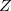Q-Chem 5.0 User’s Manual

# 9.5 Intrinsic Reaction Coordinate

The concept of a reaction path is chemically intuitive (a pathway from reactants to products) yet somewhat theoretically ambiguous because most mathematical definitions depend upon the chosen coordinate system. Stationary points on a potential energy surface are independent of this choice, but the path connecting them is not, and there exist various mathematical definitions of a “reaction path”. Q-Chem uses the intrinsic reaction coordinate (IRC) definition, as originally defined by Fukui , which has come to be a fairly standard choice in quantum chemistry. The IRC is essentially sequence of small, steepest-descent paths going downhill from the transition state.

The reaction path is most unlikely to be a straight line and so by taking a finite step length along the direction of the gradient you will leave the “true” reaction path. A series of small steepest descent steps will zig-zag along the actual reaction path (a behavior known as “stitching”). Ishida et al.  developed a predictor-corrector algorithm, involving a second gradient calculation after the initial steepest-descent step, followed by a line search along the gradient bisector to get back on the path, and this algorithm was subsequently improved by Schmidt et al. . This is the method that Q-Chem adopts. It cannot be used for the first downhill step from the transition state, since the gradient is zero, so instead a step is taken along the Hessian mode whose frequency is imaginary.

The reaction path can be defined and followed in-matrix coordinates, Cartesian coordinates or mass-weighted Cartesian coordinates. The latter represents the “true” IRC as defined by Fukui . If the rationale for following the reaction path is simply to determine which local minima are connected by a given transition state, which, is arguably the major use of IRC algorithms, then the choice of coordinates is irrelevant. In order to use the IRC code, the transition state geometry and the exact Hessian must be available. These must be computed via two prior calculations, with JOBTYPE = TS (transition structure search) and JOBTYPE = FREQ (Hessian calculation), respectively. Job control variables and examples appear below.

An IRC calculation is invoked by setting JOBTYPE = RPATH in the \$rem section, and additional \$rem variables are described below. IRC calculations may benefit from the methods discussed in Section 9.2 for obtaining good initial guesses for transition-state structures.

RPATH_COORDS
 Determines which coordinate system to use in the IRC search.

TYPE:
 INTEGER

DEFAULT:
 0

OPTIONS:
 0 Use mass-weighted coordinates. 1 Use Cartesian coordinates. 2 Use Z-matrix coordinates.

RECOMMENDATION:
 Use the default.

RPATH_DIRECTION
 Determines the direction of the eigenmode to follow. This will not usually be known prior to the Hessian diagonalization.

TYPE:
 INTEGER

DEFAULT:
 1

OPTIONS:
 1 Descend in the positive direction of the eigen mode. -1 Descend in the negative direction of the eigen mode.

RECOMMENDATION:
 It is usually not possible to determine in which direction to go a priori, and therefore both directions will need to be considered.

RPATH_MAX_CYCLES
 Specifies the maximum number of points to find on the reaction path.

TYPE:
 INTEGER

DEFAULT:
 20

OPTIONS:User-defined number of cycles.

RECOMMENDATION:
 Use more points if the minimum is desired, but not reached using the default.

RPATH_MAX_STEPSIZE
 Specifies the maximum step size to be taken (in 0.001 a.u.).

TYPE:
 INTEGER

DEFAULT:
 150 corresponding to a step size of 0.15 a.u..

OPTIONS:Step size =/1000 a.u.

RECOMMENDATION:
 None.

RPATH_TOL_DISPLACEMENT
 Specifies the convergence threshold for the step. If a step size is chosen by the algorithm that is smaller than this, the path is deemed to have reached the minimum.

TYPE:
 INTEGER

DEFAULT:
 5000 Corresponding to 0.005 a.u.

OPTIONS:User-defined. Tolerance =/1000000 a.u.

RECOMMENDATION:
 Use the default. Note that this option only controls the threshold for ending the RPATH job and does nothing to the intermediate steps of the calculation. A smaller value will provide reaction paths that end closer to the true minimum. Use of smaller values without adjusting RPATH_MAX_STEPSIZE, however, can lead to oscillations about the minimum.

RPATH_PRINT
 Specifies the print output level.

TYPE:
 INTEGER

DEFAULT:
 2

OPTIONS:RECOMMENDATION:
 Use the default, as little additional information is printed at higher levels. Most of the output arises from the multiple single point calculations that are performed along the reaction pathway.

Example 9.217

```\$molecule
0  1
C
H  1  1.20191
N  1  1.22178  2  72.76337
\$end

\$rem
JOBTYPE    freq
BASIS      sto-3g
METHOD     hf
\$end

@@@

\$molecule